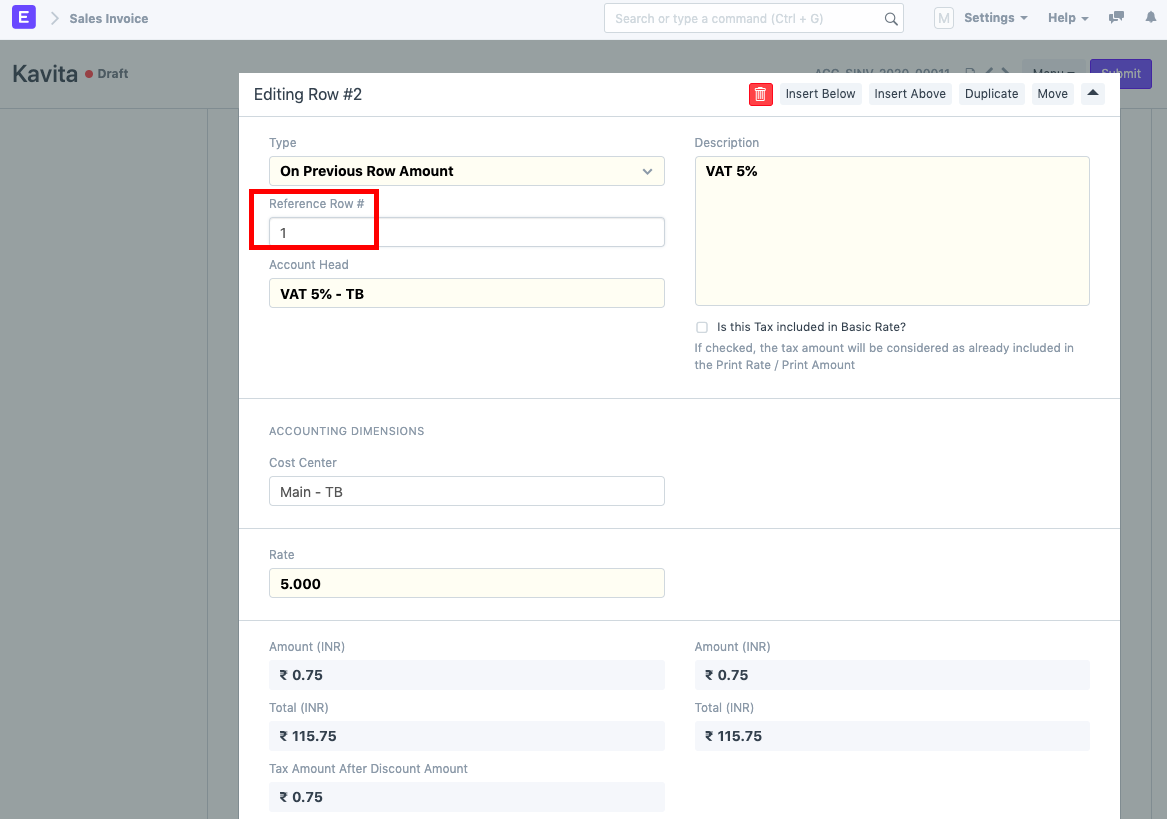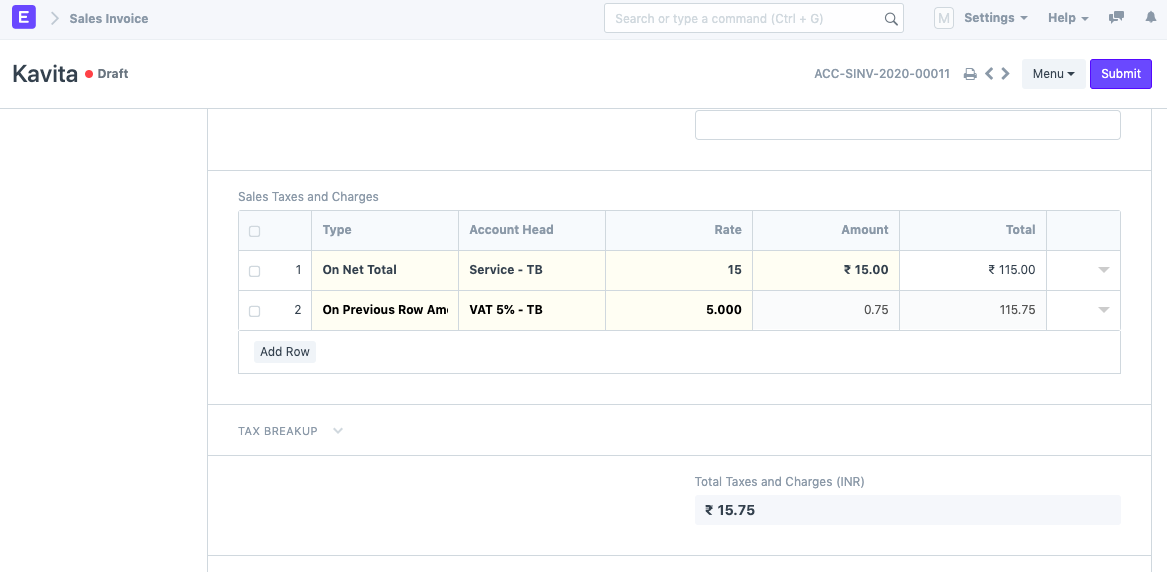###### By Michelle Alva on February 17, 2020
Intermediate
Use Case: Need to calculate tax on the previous tax amount and not on the item amount.

For example, we have 5 items and Service Charge is calculated on the Net Total of the 5 items. Additionally, we need to calculate 5% VAT on the Service Charge of the Items and not on the Net Total of the Invoice. In this case, you need to follow the following steps:

1) In Sales Invoice, under the Sales Taxes and Charges section you need to set the tax calculation. In row 1, select the Type as "On Net Total" and Account Head as needed. Enter the Rate if not set already.
The amount for this particular account head is calculated under the Amount column.

2) Next, you need to calculate tax on the previous row's amount (which is the tax amount). To do this, select the Type as "On Previous Row Amount". Set the Account Head and Rate as needed. Expand the row and set the Reference Row # as shown below.The Sales Taxes and Charges section set looks as follows: